Blog

New Course: Extreme Formulas in Google Sheets!The newest premium training course from The Collins School Of Data, Extreme Formulas in Google Sheets, is now available.

It’s all about formulas, formulas, formulas!

The course is perfect for you if:

• You enjoyed the Advanced Formulas 30 Day Challenge course
• You’re intellectually curious and enjoy solving problems
• You want to learn some crazy, cutting-edge formula techniques in Google Sheets
• You enjoy the Formula Challenges in the Monday Tips emails
• You’re an intermediate to advanced user of Google Sheets

For the next 72 hours, until Thursday 25th July at midnight, this course is available for just \$49.

After that the price will increase to \$99.

Get it now and enjoy a massive saving of 50% off the regular price!

Find out more details here >>

For a taste of what you’ll cover in this course, have a read of this post about the Google Sheets Formula Clock.

Google Sheets Formula Clock

Behold the Google Sheets Formula Clock, a working analog clock built with a single Google Sheets formula:

It’s a working analog clock built with a single Google Sheets formula.

That’s right, just a single formula. No Apps Script code. No widgets. No hidden add-ons.

Just a plain ol’ formula in Google Sheets!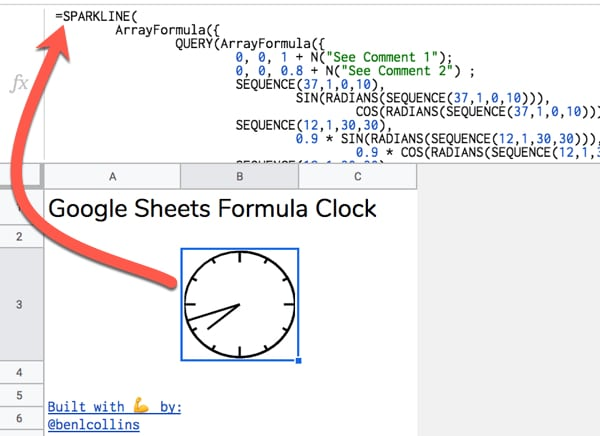Google Sheets Formula Clock Template

Click here to open the Google Sheets Formula Clock Template

(Click to open the template. Feel free to create your own copy through the File menu: File > Make a copy...)

It might take a moment to update to the current time.

Part 1: Build your own Google Sheets Formula Clock

Step 1

Open a blank Google Sheet or create a new Google Sheet

(Pro-tip: type sheet.new into your browser address bar to do this instantly)

Step 2

Copy the Google Sheets Formula Clock formula below and paste it into the formula bar for cell A1 of your new Sheet:

=SPARKLINE(
ArrayFormula({
QUERY(ArrayFormula({
0, 0, 1 + N("See Comment 1");
0, 0, 0.8 + N("See Comment 2") ;
SEQUENCE(37,1,0,10),
COS(RADIANS(SEQUENCE(37,1,0,10))) + N("See Comment 3") ;
SEQUENCE(12,1,30,30),
0.9 * COS(RADIANS(SEQUENCE(12,1,30,30))) + N("See Comment 4") ;
SEQUENCE(12,1,30,30),
COS(RADIANS(SEQUENCE(12,1,30,30))) + N("See Comment 5") ;
SEQUENCE(4,1,90,90),
0.8 * COS(RADIANS(SEQUENCE(4,1,90,90))) + N("See Comment 6") ;
SEQUENCE(4,1,90,90),
COS(RADIANS(SEQUENCE(4,1,90,90))) + N("See Comment 7")
}),
"SELECT Col2, Col3 ORDER BY Col1",
0 + N("See Comment 8")
) ;
IF(
MINUTE(NOW()) = 0,
0,
),
IF(
MINUTE(NOW())=0,
1,
) + N("See Comment 9");
0, 0 + N("See Comment 10") ;
0.75 * SIN(RADIANS((MOD(HOUR(NOW()),12)/12 * 360) + MINUTE(NOW())/60 * 30)),
0.75 * COS(RADIANS((MOD(HOUR(NOW()),12)/12 * 360) + MINUTE(NOW())/60 * 30)) + N("See Comment 11")
}),
{"linewidth",2 + N("See Comment 12")
+ N("
1: Initial (0,1) coordinate at top of circle. Extra 0 included for sort.
2: Coordinates to create mark at 12 o'clock.
3: Coordinates to draw initial circle. Joins markers every 10 degrees starting from 0 at top of circle, e.g. 0, 10, 20, 30,...360
4: Sequence of coordinates every 30 degrees to create small markers for hours 1, 2, 4, 5, 7, 8, 10, 11
5: Sequence of coordinates to connect the 30 degree small markers. Needed to place them correctly on circle.
6: Sequence of coordinates every 90 degrees to create large markers for hours 12, 3, 6, 9
7: Sequence of coordinates to connect the 90 degree large markers. Needed to place them correctly on circle.
8: QUERY function used to sort the circle data by the degrees column, then select just the (x,y) coordinate columns (numbers 2 and 3) to use.
9: Coordinates to create the minute hand. Includes an IF statement to avoid an error when the minute hand arrives at the 12 mark.
10: Coordinates to return to centre of clock at (0,0) after minute hand, to be ready to draw hour hand.
11: Coordinates to create the hour hand.
12: Set linewidth of the Sparkline to 2.
.
.
Google Sheets Formula Clock
June 2019
Created by Ben Collins, Google Developer Expert and Founder of The Collins School Of Data
Website: benlcollins.com
"
)}
)

Initially it will look like this: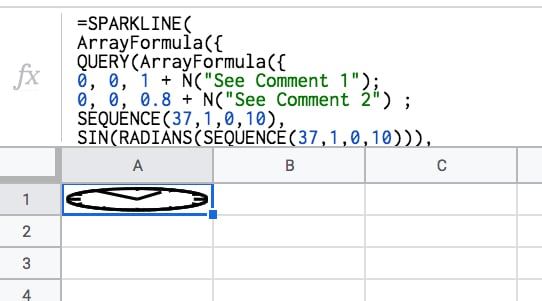Step 3

Make row 1 wider by hovering between rows 1 and 2 and using the grab hand to drag the row boundary down. Make the cell wide enough to create a circle: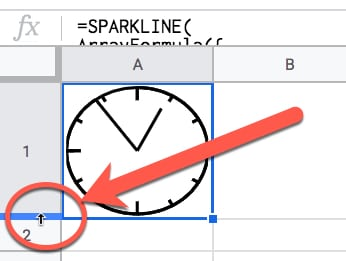Step 4

This is the step that makes the clock tick!

Under File > Spreadsheet settings set the spreadsheet calculation settings to be “On change and every minute”, like so: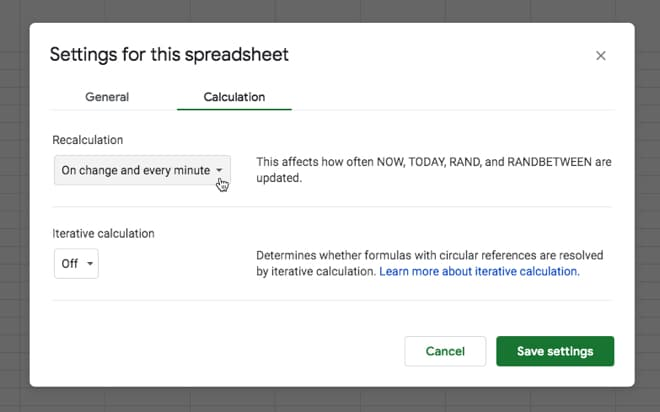This ensures that the NOW function is refreshed every minute, so our clock hands move around the circle. That’s it!

You should see the hands of your clock moving around the face.

Tick-tock! Tick-tock! 🕰️

Part 2: How Does It Work?

So there’s a few things going on here.

We need a way to get the current hour and minute values and have them update automatically.

Then somehow we need to draw a clock face with hands using…formulas? Huh? 🤔

Let’s run through the building blocks…

We can use our friend the SPARKLINE function to create the clock face:

Create A Circle With The Sparkline Function

The SPARKLINE function is used to create miniature charts inside a single cell. That’s its modus operandi.

However, we can also supply it with a range of x- and y-coordinates to create 2-d shapes, like a circle for example.

Use the following five steps to create a circle with a sparkline:

1) Start with this function in cell A1:

= SEQUENCE ( 37, 1, 0, 10 )

The Sequence function syntax is SEQUENCE(rows, columns, start, step) so in this example we’re creating 37 rows in a single column, starting from 0 and increasing in increments of 10 each.

I.e. it outputs a column of numbers representing every 10 degrees of a circle, up to 360 degrees.

2) In column B, we add this Array Formula in cell B1:

= ArrayFormula ( SIN ( RADIANS ( \$A\$1:\$A\$37 ) ) )

3) And in column C, this one in cell C1:

= ArrayFormula ( COS ( RADIANS ( \$A\$1:\$A\$37 ) ) )

Columns B and C now give you the coordinates of a circle.

4) Let’s plug them into the SPARKLINE function in cell D1 with this function:

= SPARKLINE ( B:C )

5) Lastly, make row 1 wider to show the circle.

Boom! 💥

The SPARKLINE function draws a circle for us: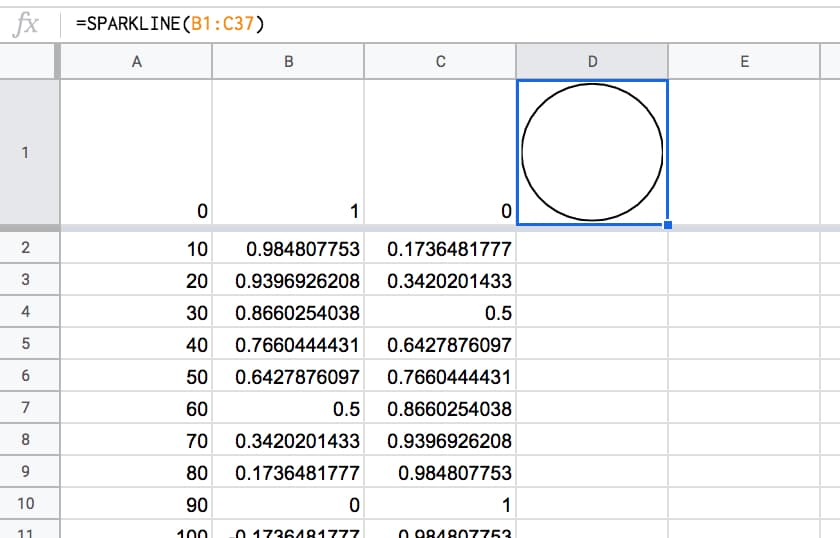Then, we need to create a time that automatically updates every minute. Thankfully that’s relatively easy to do with the NOW function:

NOW Function + Spreadsheet Settings

(Feel free to type these formulas in to the side of your sparkline workings in column B, C and D.)

= NOW()

The NOW Function in Google Sheets outputs a timestamp with a time to the nearest second. It’s a volatile function, which means it recalculates every time a change is made to the Sheet. In other words, it gives a new timestamp.

Per the Step 4 in Part 1 above, we can set the Sheet to update every minute, so the NOW function updates every minute.

Get The MINUTE And HOUR From NOW

It’s relatively easy to extract the minute and hour from the timestamp, with these two functions:

= MINUTE( NOW() )

and

= HOUR ( NOW() )

We need to convert these to degrees on a circle to show how far round the hands have gone.

The formulas become:

= MINUTE( NOW() ) / 60 * 360

and

= MOD( HOUR( NOW() ), 12 ) / 12 * 360

respectively.

Later we’ll need to convert these to RADIANS and then into coordinates for the sparkline function.

That’s the mechanics of the clock-tick-tock part, but we still need to add them to our sparkline clock.

Add The Clock Hands

The middle of our circle is represented by the coordinates (0,0).

Currently our sparkline has positioned us at the 12 o’clock position, represented by (0,1).

To add the minute hand, we need to draw another arc round the circle to travel around the edge of the circle to the current minute value, e.g. if it’s half past the hour then we need to draw another half circle to position ourselves at the bottom of the circle.

Then we can simply draw a line back to the centre of the circle, and that’s our minute hand!

So, add this function to cell B38:

=ArrayFormula( SIN ( RADIANS ( SEQUENCE ( MINUTE ( NOW( ) ) / 60 * 360 , 1 , 1 , 1 ) ) ) )

And add this one to cell C38:

=ArrayFormula( COS ( RADIANS ( SEQUENCE ( MINUTE ( NOW( ) ) / 60 * 360 , 1 , 1 , 1 ) ) ) )

Essentially, what these two formulas are doing is working out how many degrees around the circle we need to go, and calculating the coordinates.

Finally, let’s return to the centre of our circle, thereby drawing the minute hand.

In cell B398 put a 0.

In cell C398 put a 0.

They need to be on row 398 to give the array formula for the minutes enough space to expand (max 360 rows).

The “clock” now looks like this, and if you’ve set your spreadsheet to update every minute (see Step 4 in Part 1 above) then you’ll see this hand move around the clock.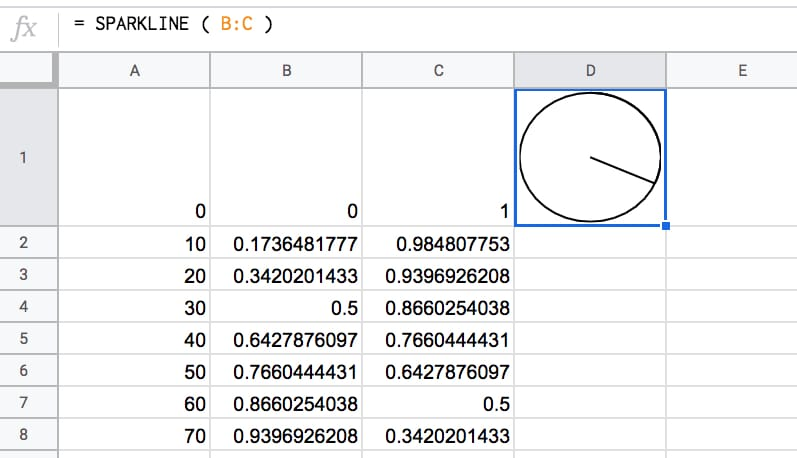To add the hour hand, it’s a case of drawing a line from the centre coordinate (0,0) — where we are now — back out to the edge, again, going as far around the circle as needed to represent the current hour.

Add this formula to cell B399:

= 0.75 * SIN ( RADIANS ( ( MOD ( HOUR ( NOW( ) ) , 12 ) / 12 * 360 ) ) )

And this formula to cell C399:

= 0.75 * COS ( RADIANS ( ( MOD ( HOUR ( NOW( ) ) , 12 ) / 12 * 360 ) ) )

This adds the hour hand.

The 0.75 multiplier at the front of the formula shortens the hour hand a little to distinguish it from the second hand.

Boom!

Now you have a working clock: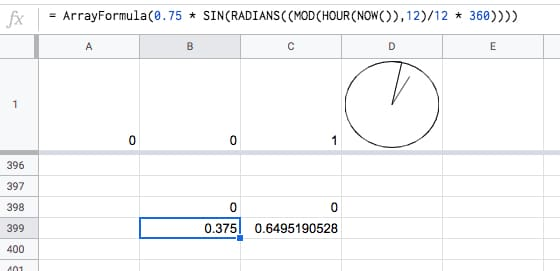Fix The Hour Issue

Unfortunately, in it’s current state, the formula breaks down at the top of the hour: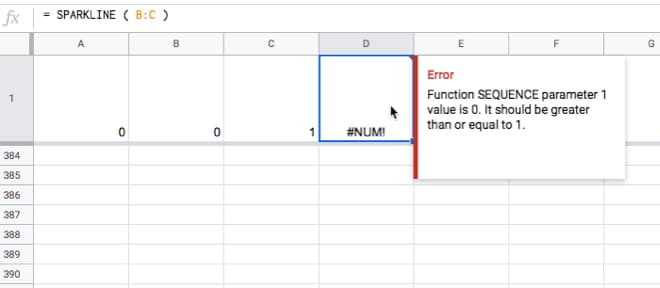This is easily solved by wrapping the minute hand calculation with an IF statement to set it to zero at the top of the hour. This IF statement tests to see if the minute component of NOW is equal to zero and sets the value to 0 if it is, otherwise we just proceed with the full SEQUENCE function.

Change the formula in cell B38 to

=ArrayFormula( IF( MINUTE( NOW() ) = 0 , 0 , SIN( RADIANS( SEQUENCE( MINUTE( NOW() ) / 60*360 , 1 , 1 , 1 )))))

and the formula in cell C38 to

=ArrayFormula( IF( MINUTE( NOW() ) = 0 , 0 , COS( RADIANS( SEQUENCE( MINUTE( NOW() ) / 60*360 , 1 , 1 , 1 )))))

It won’t look any different but you’ll avoid that error when the minute hand goes past the hour mark.

This formula is demonstrated in tab 2 of the intermediary template.

The clock will now look something like this: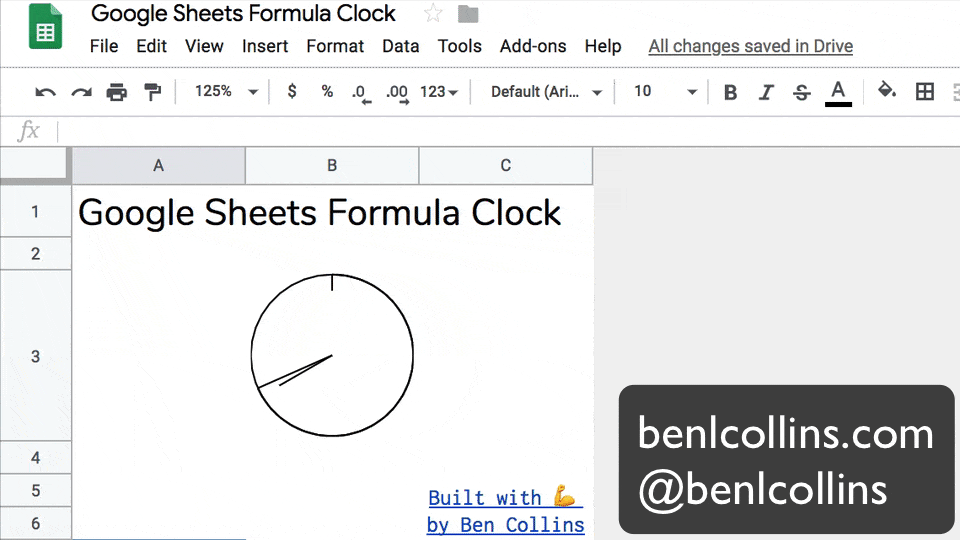So what’s left?

Improvements

You might consider the following improvements, but I’ll leave these as a challenge for you:

• Smoothing the hour hand, so it doesn’t jump in discrete steps from hour to hour but instead moves smoothly between the hours in proportion to the number of minutes passed. (See the GIF image at the start of this post.)
• Adding tick marks at each of the 12 hour marks around the clock face.
• Combining all the separate formulas into a single array formula. Hint: you need to make use of curly brackets { } to combine the array outputs from the constituent formulas.

Implementing all of these is a little tricky, not the least because the formula gets rather long!

The best approach is to build in steps, employing the Onion Method technique to avoid frustrating errors.

Hickory, dickory, dock.
The mouse ran up the sparkline clock.
The sparkline clock struck one,
The mouse ran down,
Hickory, dickory, dock. 🐁⏱️

What Else Can You Draw With Sparklines?

How about an outline of the Saturn V rocket?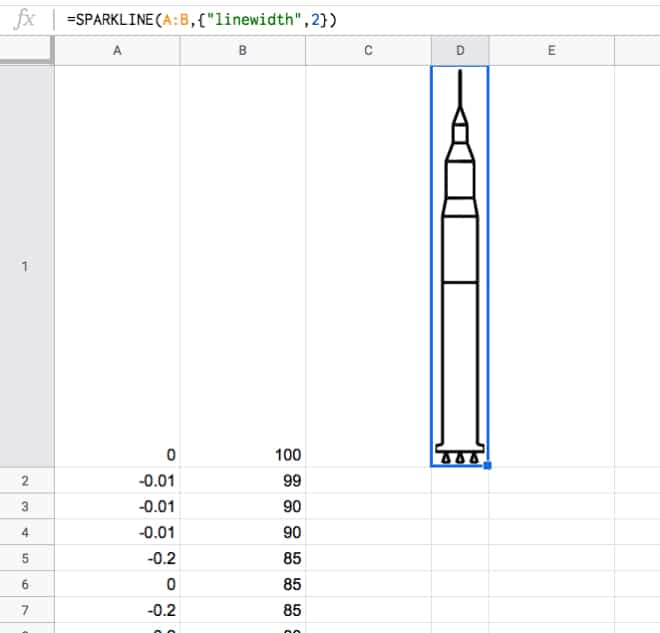Or a pie chart built with a single sparkline array formula?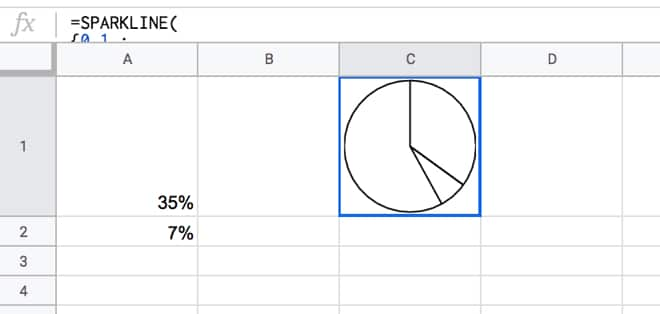This pie chart actually inspired the analog clock…you can probably see why!

18 Google Sheets Formula Tips & Techniques You Should Know

How many of these Google Sheets Formula Tips & Techniques do you know?

1. F4 Key

Undoubtedly one of the most useful Google Sheets formula shortcuts to learn.

Press the F4 key to toggle between relative and absolute references in ranges in your Google Sheets formulas.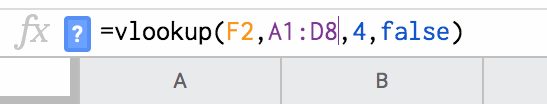It’s WAY quicker than clicking and typing in the dollar (\$) signs to change a reference into an absolute reference.

2. F2 To Edit Cell

Have you ever found yourself needing to copy part of a Google Sheets formula to use elsewhere? This is a shortcut to bring up the formula in a cell.

Start by selecting a cell containing a formula.

Press the F2 key to enter into the formula: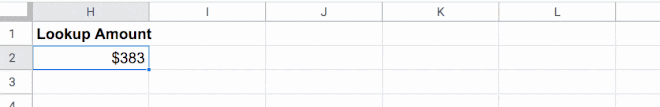3. Shift + Enter To Edit Cell

Shift + Enter is another shortcut to enter into the Google Sheets formula edit view.

4. Escape To Exit A Formula

Have you ever found yourself trying to click out of your formula, but Sheets thinks you want to highlight a new cell and it messes up your formula?

Press the Escape key to exit the formula view and return to the result view.

Any changes are discarded when you press the Escape key (to save changes you just hit the usual Return key).

5. Move To The Front Or End Of Your Google Sheets Formula

Here’s another quick trick that’s helpful for longer Google Sheets formulas:

When you’re inside the formula view, press the Up arrow to go to the front of your formula (in front of the equals sign).

Similarly, pressing the Down arrow takes you to the last character in your formula.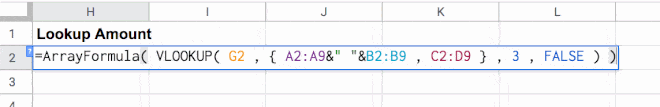6. Function Helper Pane

Learn to read the function helper pane!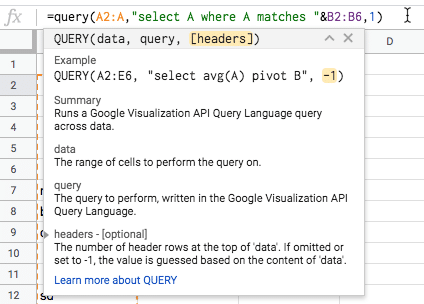You can press the “X” to remove the whole pane if it’s getting it the way. Or you can minimize/maximize with the arrow in the top right corner.

The best feature of the formula pane is the yellow highlighting it adds to show you which section of your function you are in. E.g. in the image above I’m looking at the “[headers]” argument.

There is information about what data the function is expecting and even a link to the full Google documentation for that function.

If you’ve hidden the function pane, or you can’t see it, look for the blue question mark next to the equals sign of your formula. Click that and it will restore the function helper pane.

7. Colored Ranges

Helpfully Google Sheets highlights ranges in your formulas and in your actual Sheet with matching colors. It applies different colors to each unique range in your formula.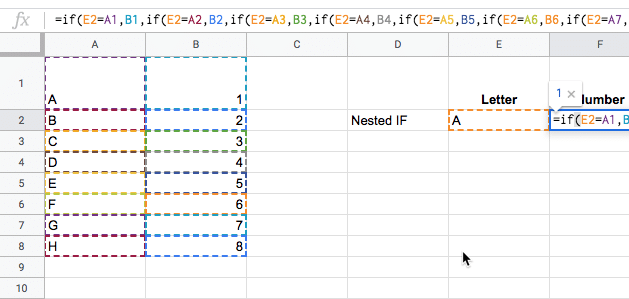8. F2 To Highlight Specific Ranges

As mentioned in Step 2, you press the F2 key to enter the formula view of a cell with a formula in.

However, it has another useful property. If you position your cursor over a range of data in your formula and then press the F2 key, it will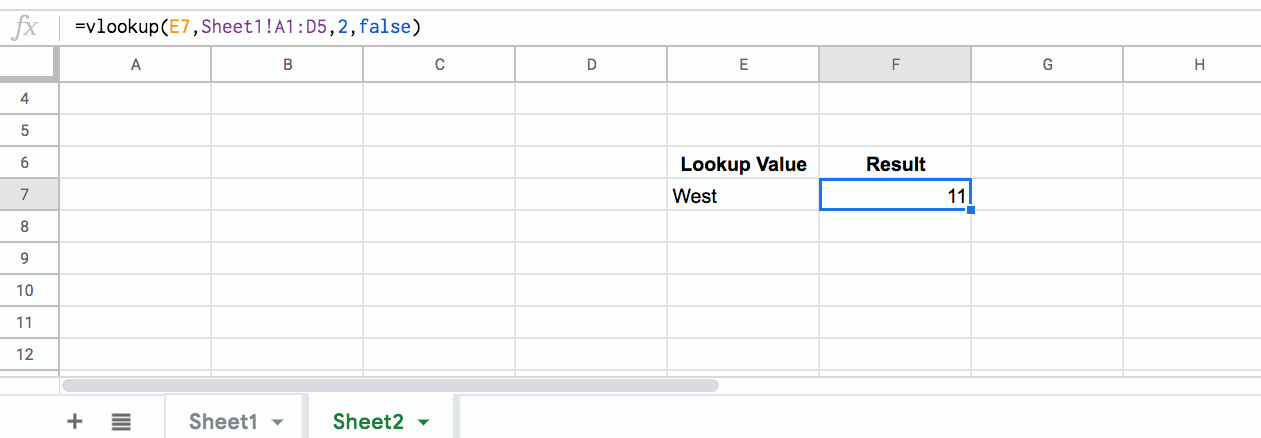9. Function Name Drop-Down

A great way to discover new functions is to simply type a single letter after an equals sign, and then browse what comes up: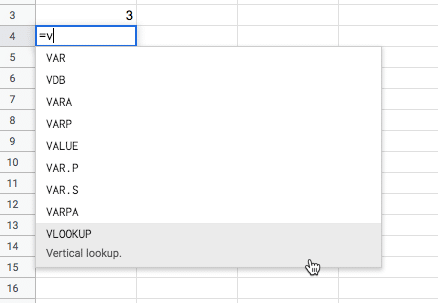Scroll up and down the list with the Up and Down arrows, and then click on the function you want.

10. Tab To Auto-Complete Function Name

When you’re using the function drop-down list in the tip above, press the tab key to auto-complete the function name (based on whatever function is highlighted).

11. Adjust The Formula Bar Width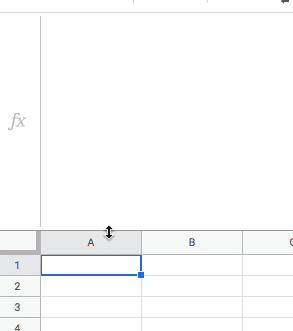An easy one this! Grab the base of the formula bar until you see the cursor change into a little double-ended arrow. Then click and drag down to make the formula bar as wide as you want.

12. Quick Aggregation Toolbar

Highlight a range of data in your Sheet and check out the quick aggregation tool in the bottom toolbar of your Sheet (bottom right corner).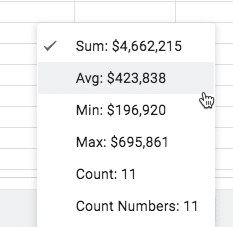Quickly find out the aggregate measures COUNT, COUNT NUMBERS, SUM, AVERAGE, MIN and MAX, without needing to create functions.

13. Quick Fill Down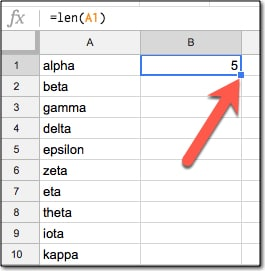To copy the formula quickly down the column, double-click the blue mark in the corner of the highlighted cell, shown by the red arrow. This will copy the cell contents and format down as far as the contiguous range in preceding column (column A in this case).

An alternative way to quickly fill in a column is to highlight the range you want to fill, e.g.: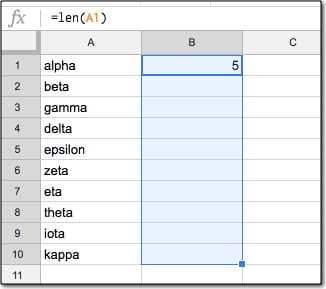Then press Ctrl + D (PC and Chromebook) or Cmd + D (Mac) to copy the contents and format down the whole range, like so: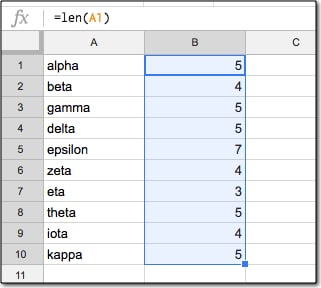You can also do this with Ctrl + Enter (PC and Chromebook) or Cmd + Enter (Mac), which will fill down the column.

14. Know How To Create An ArrayFormula

Array Formulas are powerful extensions to regular formulas, allowing you to work with ranges of data rather than individual pieces of data.

Per the official definition, array formulas enable the display of values returned into multiple rows and/or columns and the use of non-array functions with arrays.

In a nutshell: whereas a normal formula outputs a single value, array formulas output a range of cells!

We need to tell Google Sheets we want a formula to be an Array Formula. We do this in two ways:

1. Hit Ctrl + Shift + Enter (PC/Chromebook) or Cmd + Shift + Enter (on a Mac) and Google Sheets will add the ArrayFormula wrapper
2. Alternatively, type in the word ArrayFormula and add brackets to wrap your formula/li>

15. Create Arrays With Curly Brackets

Have you ever used the curly brackets, or ARRAY LITERALS to use the correct nomenclature, in your formulas?

An array is a table of data. They can be used in the same way that a range of rows and columns can be used in your formulas. You construct them with curly brackets: { }

Commas separate the data into columns on the same row.

Semi-colons creates a new row in your array.

(Please note, if you’re based in Europe, the syntax is a little different. Find out more here.)

This formula, entered into cell A1, will create a 2 by 2 array that puts data in the range A1 to B2:

= { 1 , 2 ; 3 , 4 }

The array component (in this example { 1 , 2 ; 3 , 4 } ) can be used as an input to other formulas.

16. Multi-line Formulas

Press Ctrl + Enter inside the formula editor bar to add new lines to your formulas, to make them more readable. Note, you’ll probably want to widen the formula bar first, per tip 11.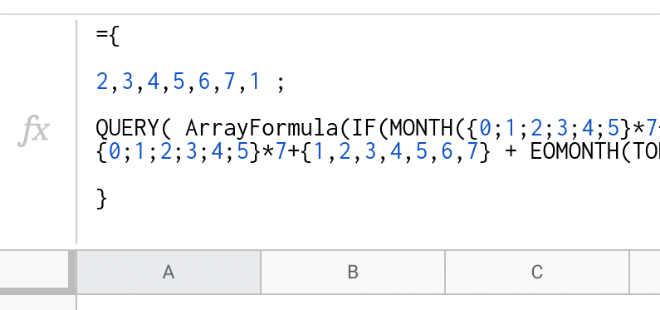17. Comments In Formulas

N returns the argument provided as a number. If the argument is text, inside quotation marks, the N function returns 0.

So we can use it to add a comment like this:

=SUM(A1:A100) + N("Sums the first 100 rows of column A")

which is effectively the same as:

=SUM(A1:A100) + 0

which is just:

=SUM(A1:A100)

This tip is pretty esoteric, but it’s helpful for any really long formulas!

18. Use The Onion Approach For Complex Formulas

Complex formulas are like onions on two counts: i) they have layers that you can peel back, and ii) they often make you cry 🤣

Use The Onion Method To Approach Complex Formulas

If you’re building complex formulas, then I advocate a one-action-per-step approach. What I mean by this is build your formula in a series of steps, and only make one change with each step. So if you start with function A(range) in a cell, then copy it to a new cell before you nest it with B(A(range)), etc.

This lets you progress in a step-by-step manner and see exactly where your formula breaks down.

Similarly, if you’re trying to understand complex formulas, peel the layers back until you reach the core (which is hopefully a position you understand). Then, build it back up in steps to get back to the full formula.

For more detail about this approach, including examples and worksheets for each case, have a read of this post:

Use The Onion Method To Approach Complex Formulas

If Google Sheets And Microsoft Excel Went For A Drink…

This is a transcript of a conversation between two famous spreadsheet applications, Google Sheets and Microsoft Excel, who, in mid-2019, sat down together at a well-known beach bar, The Pivot & Chart Tavern, for a catch-up after a long WORKDAY.

For the DURATION of their meeting, SMALL and LARGE FISHERmen came and went, smelling of POISSON from the Sea.

It was a DAY to remember.

Google Sheets: “Excel! Dude! GAUSS who, yo? It’s been DAYS, MONTHs even, since we caught up. You made it. You crash so often I wasn’t sure you’d get here.”

XL: “Ah Google Sheets, you again. Rude, impetuous, cheeky. I see you’re still as mature as toddler in a COT. Still on a formula-only diet are you? Do you know FACT from fiction yet? Is this establishment to your satisfaction? I do hope it’s NOT out of your PRICE range.”

Sheets: “Woah, so aggressive. Nope, I’m all grown up now. IMREAL deal! I’m TRIM, check out my ABS. Still TRENDy and UNIQUE of course. I have so much going on right NOW, so many cool and COMPLEX features, and an ever growing, engaged, passionate community.

What about you, Excel, still hanging on? Haha.”

XL: “Hanging on? Totally FALSE! You should respect your elders.

NOW listen to me young man, I was doing advanced financial modeling whilst you were still popping zits on your funny little (inter)face. I may be over 30 YEARs old but I’m in the best health I’ve ever been. I continue to enjoy consistent product GROWTH.

Contrary to some of the marketing materials new-fangled upstarts put out, I’m very much alive and kicking, and still dominating the office data analytics scene, thank you very much. It seems you’re in rude health too Sheets, your voice is LOWER NOW, full of CONFIDENCE. Let me buy you a drink.”

Sheets: Sure, a beer please.

XL: So unsophisticated.

Turning to the barman…

XL: A beer, and I’ll have your finest aged red wine please. Put it all on the same TAB, thank you.

Barman: That’ll be 0.00091 Bitcoin please.

XL: Oh come on! Can you convert that TO_DOLLARS please?

Barman: As you CHOOSE, let me SWITCH the payment….that’ll be 10 Dollars EVEN at TODAY‘s price…

Excel hands over a 20 Dollar bill.

Barman: Is that DMIN bill you’ve got?

XL: Yes, I’m sorry for the trouble.

Barman: Ok, DMAX change I have is in 1 Dollar bills…

XL: That is no problem.

After a short SEARCH for an AREA to sit, and a brief interruption when they were INTERCEPTed by an errant ROMAN soldier, they took their seats at one of the PIVOT TABLES near the bar, to continue their rather KURT conversation…

Sheets: Do you think ISODD Excel? I MEAN, here we are in rude health, still the pre-eminent way the majority of knowledge workers manage and analyze their data.

XL: Yes, it’s TRUE! We have some sticking POWER that’s for sure. I take it as a good SIGN that our respective platforms continue to evolve and maintain their critical usefulness.

Sheets: Ok, let’s get down to business then. I want to share my theory of why we’re still the pre-eminent solution for many people…

XL: Ok, Sheets, the FLOOR is yours:

Sheets: First off, we’re ubiquitous. We’re everywhere. You’re in every office and I’m in every browser. So there’s that.

Second, we can solve most problems. Yes, there is ultra-specific software that will do certain tasks better, but nobody beats us for overall utility.

Third, we’re easy to use. Beginners can just dive right in, but we’re complex enough that even the most advanced users will never run out of things to discover.

XL: RIGHT, All TRUE, all good points. We’re definitely on the same FREQUENCY here.

Sheets: AND, we’re super flexible, so we can easily adapt to new tasks or new use cases.

XL: Yes, yes, indeed. Plus, almost all SaaS platforms have a button that exports data to Excel or Sheets. I suspect a lot of people use this, but of course that’s not a good metric for a SaaS company to divulge.

XL AND Sheets both have a little chuckle at this…

The conversation rambled on for several more HOURs. The EFFECT of the drinks made the conversation take an ODD turn:

XL:Have you ever BIN2OCT-oberfest, Sheets? You know the one I mean, the beer festival in Bavaria in the fall?

Sheets: Yeah, yeah I know the one, but no, I haven’t. Have you ever BIN2HEXham, XL?

XL: You mean the market town in the UK, right? Only once. And the airline lost my TRUNC on that trip! What a palava that was!

Sheets: TRUNC! Bwah! Now you’re showing your age. Haha. And definitely no chance of a TAN at that TIME of YEAR.

At a lull in the conversation, they both look down at their phones.

Sheets: Check this out, old man.

Sheets holds up his phone, with an app open called INDEX MATCH.

Sheets: It’s a dating service for spreadsheets. You right click on Sheets you like, left click on ones you don’t. It uses their IMPORTRANGE algorithm to MATCH you with other Sheets. Super cool.

XL: Bah, sounds like it’s just for HLOOKUPs to me. The more discerning spreadsheets look for love through a service called EDATE, all based around your star SIGN.

Sheets: Sounds like hokum to me…

You hungry Excel? Shall we get a PI?

Excel: You mean like a pizza PI? Could do, as long as we ADD spinach and ricotta, MINUS the mushrooms. Though I’d rather have CHAR-grilled steak.

Later, replete after dinner, it was time for the two friends to bid farewell…

XL: Right then Sheets, before you SLOPE off, let me tell you, it was good to catch up. TEXT me whenever you want to have a drink together again.

Sheets: ISEMAIL ok?

XL: As you wish. I’ll ask Numbers, LibreOffice, Airtable and maybe a few others to JOIN us next time, ok? They’re PROBably feeling LEFT out.

Sheets: Yep, I’ll be there. Catch up soon!

It certainly was a DAY to remember.

Resources

The full list of 400+ functions in Google Sheets

My rather silly story was inspired by a similar, although much funnier, function-themed story from Mr Spreadsheet himself, John Walkenbach. Sadly I can’t find it online anymore, but if anyone can share the link, I’ll add it here.

Use The Onion Method To Approach Complex Formulas

Complex Formulas? The Onion Method? Huh?

I’m talking about the idea that complex formulas in Google Sheets are a lot like onions.

They have layers.

And they sometimes make you cry. 🤣

The Onion Method For Complex Formulas

If you’re building complex formulas, then I advocate following a one-action-per-step approach.

What I mean by this is that you build your formulas in a series of steps, and only make one change with each step.

The Onion Method is a framework by which to approach hard formulas, and consists of these three elements:

1. Put each new step of the formula in a new cell
2. Label each step with a simple “Step 1”, “Step 2”, etc. in adjacent cells
3. Change the background color of each formula cell, so they can be easily found

This lets you see the formula progress in an incremental way and is really helpful when you’re building or tyring to understand complex formulas.

Sometimes a step might result in an error (typically a #N/A or #REF!), but that’s ok, provided it gets fixed in a subsequent step, as shown in this SUMPRODUCT example: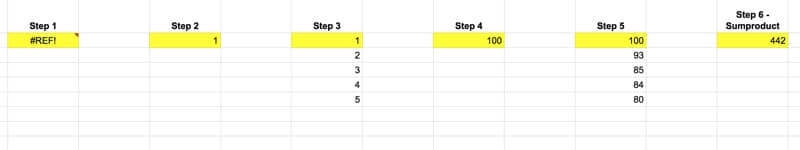Each of these intermediary formulas in the above image moves us forward incrementally, until the final answer is obtained in step 6.

Similarly, if you’re trying to understand complex formulas, peel the layers back until you reach the core (which is hopefully a function you understand!). Then, build it back up in steps to get back to the full formula.

Example 1: Building A Complex Formula With The Onion Method

Let’s look at importing data from the table on this Wikipedia page of largest cities by population.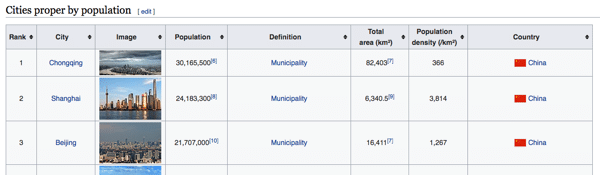Step 0

Open a new Google Sheet (bonus points for using the browser shortcut and just typing Sheets.new into your browser window).

Step 1

Step 1 is a standard IMPORTHTML function to retrieve the city table from Wikipedia. In cell A2:

=IMPORTHTML("https://en.wikipedia.org/wiki/List_of_cities_proper_by_population","table",2)

The data has some issues, but it’s a start.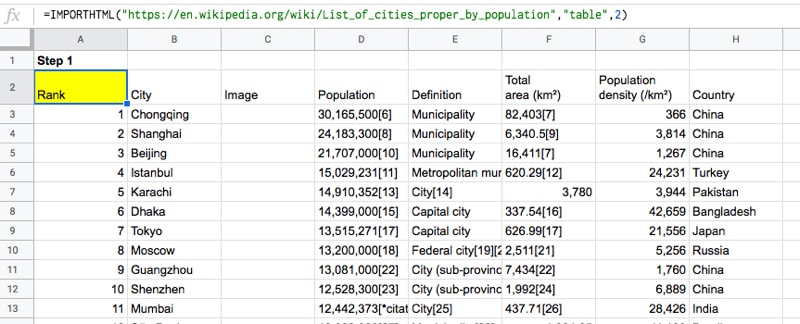Step 2

In Step 2, we use the INDEX function to grab just the population column.

Per The Onion Method, we insert this next step in a new cell, cell J2, to the right of the existing data, in this example:

=INDEX(IMPORTHTML("https://en.wikipedia.org/wiki/List_of_cities_proper_by_population","table",2),,4)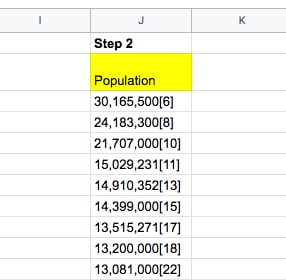I’ve highlighted the new action step in red — adding the INDEX function wrapper.

Looking closely at that INDEX function wrapper, you’ll see that we’ve left the row argument blank, namely:

INDEX(data, ,4)

which returns the entire column.

We’ll deal with the population column on its own and come back to our main formula later.

Step 3

Hmm, that population column is messed up! Regex to the rescue!

Using the REGEXEXTRACT function, extract just the numbers and “,” from the data, before the citations in brackets:

=REGEXEXTRACT(INDEX(IMPORTHTML("https://en.wikipedia.org/wiki/List_of_cities_proper_by_population","table",2),,4),"[0-9,]+")

Hmm, that gives us a #N/A error… 🤔

Step 4

Turn this into an Array Formula and get the column of population numbers!

=ArrayFormula(REGEXEXTRACT(INDEX(IMPORTHTML("https://en.wikipedia.org/wiki/List_of_cities_proper_by_population","table",2),,4),"[0-9,]+"))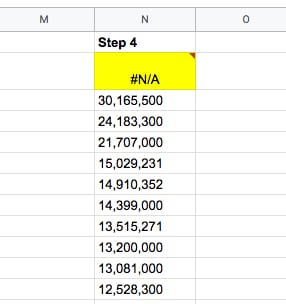We still have two problems to solve though: i) we need to convert the strings into actual numbers and ii) we need to fix the #N/A column heading…

Step 5

We can multiply by 1 (see the “*1” at the end of our formula), which coerces those text strings into actual numbers.

=ArrayFormula(REGEXEXTRACT(INDEX(IMPORTHTML("https://en.wikipedia.org/wiki/List_of_cities_proper_by_population","table",2),,4),"[0-9,]+") * 1)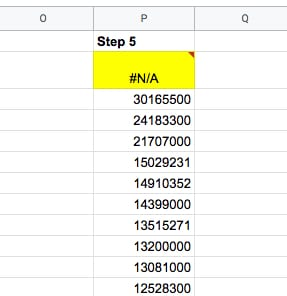Step 6

Use the IFERROR function to fix that pesky #N/A error at the top of our column heading, and replace the #N/A with the word “Population”:

=ArrayFormula(IFERROR(REGEXEXTRACT(INDEX(IMPORTHTML("https://en.wikipedia.org/wiki/List_of_cities_proper_by_population","table",2),,4),"[0-9,]+")*1,"Population"))

Nice, now we have our population column as numbers:Step 7

Pick the other columns we want, by wrapping the IMPORTHTML function with a QUERY function.

Note that we have to use the Col1 notation rather than the column letter in our Select statement, since we’re nesting another function as our data source in the QUERY function.

We also use the QUERY function rather than the INDEX function because we want to return multiple columns this time, which the INDEX function can’t do.

=QUERY(IMPORTHTML("https://en.wikipedia.org/wiki/List_of_cities_proper_by_population","table",2),"SELECT Col2, Col8",1)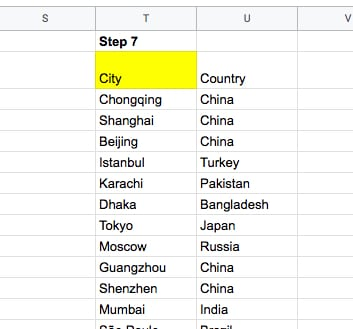Step 8

All that’s left is to join these two ranges using the curly bracket notation, like this:

={

QUERY(IMPORTHTML("https://en.wikipedia.org/wiki/List_of_cities_proper_by_population","table",2),"SELECT Col2,Col8",1)
,
ArrayFormula(IFERROR(REGEXEXTRACT(INDEX(IMPORTHTML("https://en.wikipedia.org/wiki/List_of_cities_proper_by_population","table",2),,4),"[0-9,]+") * 1,"Population"))

}

(Shown with line breaks to illustrate the two ranges.)

Ok, we’re done 🤪

The output is:Example 2: Deconstructing With The Onion Method

If you’re trying to understand complex formulas in Google Sheets that someone else has shared with you, you can still approach it with this Onion Method.

Simply peel back the layers until you reach the innermost function. Copy that into a new cell and start from the inside and work out, building up to the full formula again.

Let’s see an example.

Suppose we’re given this worksheet with US State names: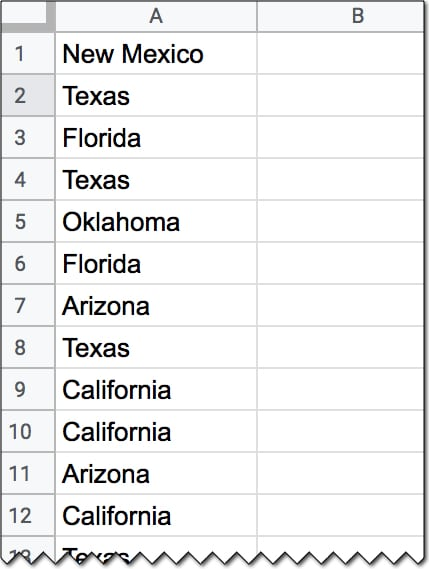And we’re also given this formula:

=ArrayFormula(INDEX(A1:A20,MODE(MATCH(A1:A20,A1:A20,0))))

which gives an output of Texas.

But how does this formula work?

Applying The Onion Method, we peel back the layers to the core function, and then build it up in steps again.

Step 1

In a new cell, add the innermost MATCH function:

=MATCH(A1:A20,A1:A20,0)

Step 2

=ArrayFormula(MATCH(A1:A20,A1:A20,0))

which outputs an array of the position of the first occurrence of the words in column A. We see a 2 next to every occurrence of Texas for example, because the first time it occurred was in position 2.

Step 3

Now, we wrap it with the MODE function to find the most frequently occurring position:

=ArrayFormula(MODE(MATCH(A1:A20,A1:A20,0)))

By definition, the MODE function takes a range of numbers for an input and finds the most commonly occurring value.

However, what happens if we have a range of text values and want to find the most frequent?

In this case, the MATCH has been used to create a range of numbers for the MODE function.

By now, we’ve probably deduced that this formula finds the most frequent word in a list.

Step 4

Finally, we can retrieve the actual text value, i.e. the most frequent State name, by adding the INDEX function to get the full original formula, like this:

=ArrayFormula(INDEX(A1:A20,MODE(MATCH(A1:A20,A1:A20,0))))

This will give the output Texas in this specific example.

Nice!

Template For Your Use

Click here to open a read-only copy of the template >>

This template contains both examples from this tutorial.

To make your own editable copy, please go to File > Make a copy… under the File menu.

Conclusion

The Onion Method is a framework that allows you to approach complex formulas in a systematic way.

Even if you’re presented with an “impossible” challenge to answer or an “impossible” formula to decipher, just follow this framework. If required, peel back the layers and then work from the inside out in an incremental fashion.

You’ll be amazed at how quickly your understanding of challenging formulas broadens and deepens. You’ll encounter and understand brand new functions that you’ve never heard of before. Plus, you’ll find out all sorts of secret tricks with existing formulas.

Who knows, you might even cry tears of joy instead of despair…

I’ll leave you with this quote from businesswoman Belinda Johnson:

I like cutting through complexity and trying to get to the kernel of an idea.